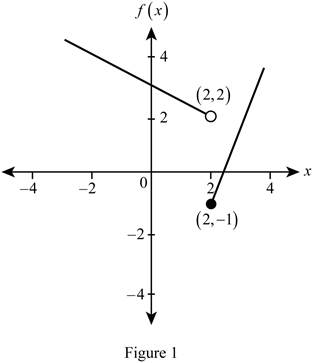# The values of f ( − 3 ) , f ( 0 ) , f ( 2 ) and sketch the graph of piecewise function.### Single Variable Calculus: Concepts...

4th Edition
James Stewart
Publisher: Cengage Learning
ISBN: 9781337687805### Single Variable Calculus: Concepts...

4th Edition
James Stewart
Publisher: Cengage Learning
ISBN: 9781337687805

#### Solutions

Chapter 1.1, Problem 44E
To determine

## To find: The values of f(−3),f(0),f(2) and sketch the graph of piecewise function.

Expert Solution

The value of f(3)=92 .

The value of f(0)=3 .

The value of f(2)=1 .

### Explanation of Solution

Given:

The function is f(x)={312xif x<22x5if x2 .

Calculation:

Obtain the values of f(3),f(0) and f(2) as follows:

Since the value of x (=3) is less than 2, substitute −3 for x in the function f(x)=312x .

f(3)=312(3)=3+32=92

The value of f(3)=92 .

Since the value of x (=0) is less than 2, substitute 0 for x in the function f(x)=312x .

f(0)=312(0)=3

The value of f(0)=3 .

Since the value of x is equal to 2, substitute 2 for x in the function f(x)=2x5 .

f(2)=2(2)5=45=1

The value of f(2)=1 .

When x<2 , f(x)=312x is a line with slope 12 and y-intercept 3 that lies to the left of the line x = 2.

When x2 , f(x)=2x5 is a line with slope 2 and y-intercept −5 that lies to the right of the line x = 2.

The graph of f(x) is shown below in Figure 1.In Figure 1, the open dot indicates that the point (2, 2) is excluded and the closed dot indicates that the point (2, −1) is included.

### Have a homework question?

Subscribe to bartleby learn! Ask subject matter experts 30 homework questions each month. Plus, you’ll have access to millions of step-by-step textbook answers!